# How does this works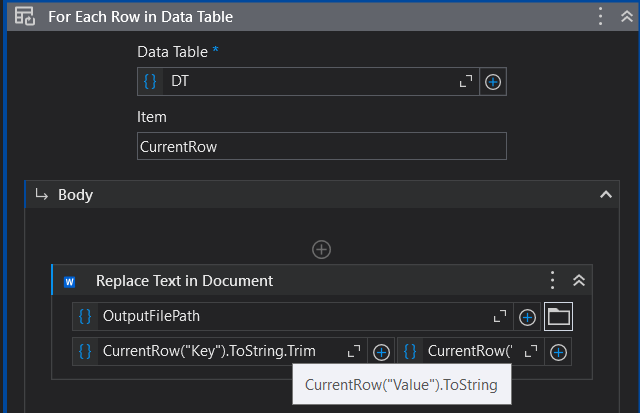Can someone explain to me how does this work, using datatable and using it like a dictionary, where key matches to values in a datatable

Hi,

Your Code is taking a datatable which seems to have dictionaries as type in there. So you replace in your following Document words which appear from the key List with the value item.

Dictionary is using key value pairs. So you have for every key-entry a value. Like [Name:Michael] (Key:Value).

Please give us more details if you need further help.

but there is no indication of it having dictionaries as type in there

Hi,

Let’s assume content of DT is as the following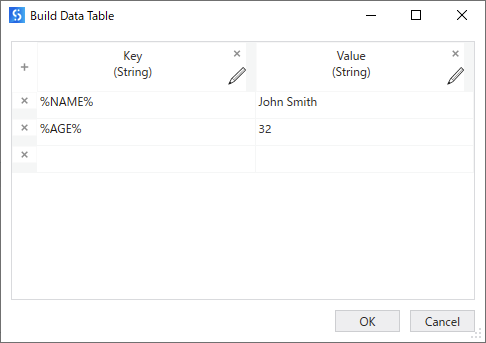And word document is as the following.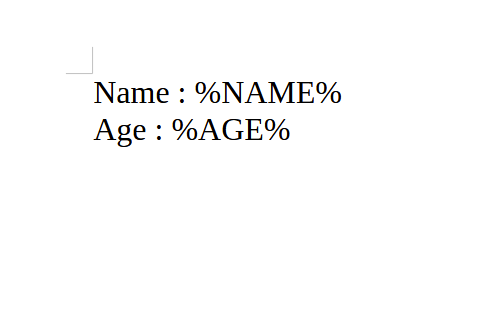In the loop, first , CurrentRow(“Key”).ToString.Trim is %NAME% nad CurrentRow(“Value”).ToString is John Smith.
So all the “%NAME%” will be replaced with “John Smith” as the following.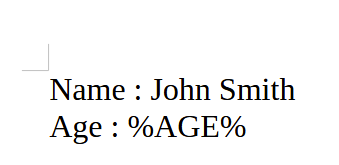Next iterate, CurrentRow(“Key”).ToString.Trim is %AGE% nad CurrentRow(“Value”).ToString is 33.
So it will be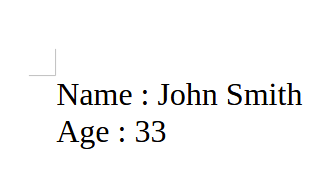Like the above, all the key string which is defined in Key column of DataTable will be replaced with value string which is defined Value column.

Regards,

2 Likes

Can you share the Value of your dt from debug-window?

I think it is stored in there.

If not, I think you have to explain the Problem some more.

Do you wrote this activity? What should it do in your opinion?

In Your DT Datatable by using for each row in datatable it will go through each row in DT

You will get the column value where header is “Key” using the currentRow(“Key”)
You are replacing it with the value field from DT Datatable.

This is the way to get the value from datatable. You can even use index insted of cloumn header.

what does the CurrentRow in item means? also what does it mean in the CurrenRow(“Key”).ToString

In datatable we store data in rows & columns like Excel. Where column has headers. In your case in datatable (DT) “Key” is the column name. When we use for each row in datatable it will go through each row in that datatable where column header is “Key”.

I hope this helps2 Likes